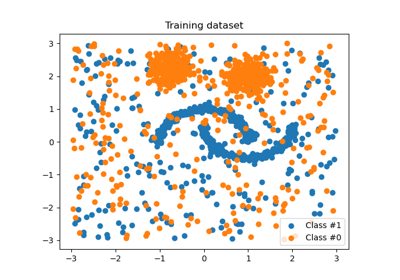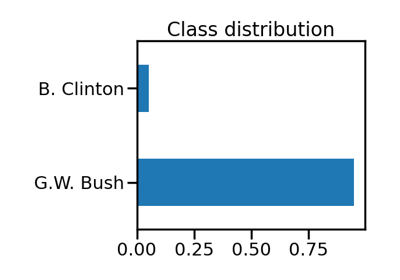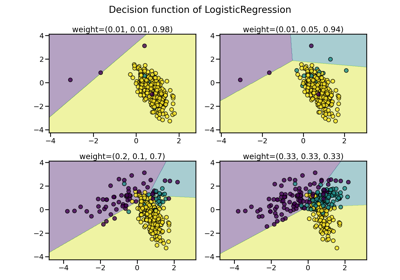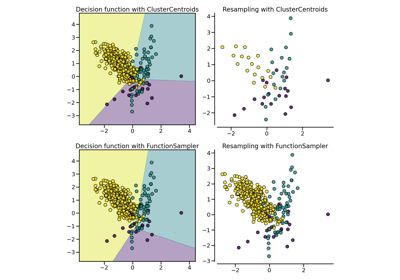# FunctionSampler#

class imblearn.FunctionSampler(*, func=None, accept_sparse=True, kw_args=None, validate=True)[source]#

Construct a sampler from calling an arbitrary callable.

Read more in the User Guide.

Parameters:
funccallable, default=None

The callable to use for the transformation. This will be passed the same arguments as transform, with args and kwargs forwarded. If func is None, then func will be the identity function.

accept_sparsebool, default=True

Whether sparse input are supported. By default, sparse inputs are supported.

kw_argsdict, default=None

The keyword argument expected by `func`.

validatebool, default=True

Whether or not to bypass the validation of `X` and `y`. Turning-off validation allows to use the `FunctionSampler` with any type of data.

New in version 0.6.

Attributes:
sampling_strategy_dict

Dictionary containing the information to sample the dataset. The keys corresponds to the class labels from which to sample and the values are the number of samples to sample.

n_features_in_int

Number of features in the input dataset.

New in version 0.9.

feature_names_in_ndarray of shape (`n_features_in_`,)

Names of features seen during `fit`. Defined only when `X` has feature names that are all strings.

New in version 0.10.

`sklearn.preprocessing.FunctionTransfomer`

Stateless transformer.

Notes

Examples

```>>> import numpy as np
>>> from sklearn.datasets import make_classification
>>> from imblearn import FunctionSampler
>>> X, y = make_classification(n_classes=2, class_sep=2,
... weights=[0.1, 0.9], n_informative=3, n_redundant=1, flip_y=0,
... n_features=20, n_clusters_per_class=1, n_samples=1000, random_state=10)
```

We can create to select only the first ten samples for instance.

```>>> def func(X, y):
...   return X[:10], y[:10]
>>> sampler = FunctionSampler(func=func)
>>> X_res, y_res = sampler.fit_resample(X, y)
>>> np.all(X_res == X[:10])
True
>>> np.all(y_res == y[:10])
True
```

We can also create a specific function which take some arguments.

```>>> from collections import Counter
>>> from imblearn.under_sampling import RandomUnderSampler
>>> def func(X, y, sampling_strategy, random_state):
...   return RandomUnderSampler(
...       sampling_strategy=sampling_strategy,
...       random_state=random_state).fit_resample(X, y)
>>> sampler = FunctionSampler(func=func,
...                           kw_args={'sampling_strategy': 'auto',
...                                    'random_state': 0})
>>> X_res, y_res = sampler.fit_resample(X, y)
>>> print(f'Resampled dataset shape {sorted(Counter(y_res).items())}')
Resampled dataset shape [(0, 100), (1, 100)]
```

Methods

 `fit`(X, y) Check inputs and statistics of the sampler. `fit_resample`(X, y) Resample the dataset. `get_feature_names_out`([input_features]) Get output feature names for transformation. Get metadata routing of this object. `get_params`([deep]) Get parameters for this estimator. `set_params`(**params) Set the parameters of this estimator.
fit(X, y)[source]#

Check inputs and statistics of the sampler.

You should use `fit_resample` in all cases.

Parameters:
X{array-like, dataframe, sparse matrix} of shape (n_samples, n_features)

Data array.

yarray-like of shape (n_samples,)

Target array.

Returns:
selfobject

Return the instance itself.

fit_resample(X, y)[source]#

Resample the dataset.

Parameters:
X{array-like, sparse matrix} of shape (n_samples, n_features)

Matrix containing the data which have to be sampled.

yarray-like of shape (n_samples,)

Corresponding label for each sample in X.

Returns:
X_resampled{array-like, sparse matrix} of shape (n_samples_new, n_features)

The array containing the resampled data.

y_resampledarray-like of shape (n_samples_new,)

The corresponding label of `X_resampled`.

get_feature_names_out(input_features=None)[source]#

Get output feature names for transformation.

Parameters:
input_featuresarray-like of str or None, default=None

Input features.

• If `input_features` is `None`, then `feature_names_in_` is used as feature names in. If `feature_names_in_` is not defined, then the following input feature names are generated: `["x0", "x1", ..., "x(n_features_in_ - 1)"]`.

• If `input_features` is an array-like, then `input_features` must match `feature_names_in_` if `feature_names_in_` is defined.

Returns:
feature_names_outndarray of str objects

Same as input features.

Get metadata routing of this object.

Please check User Guide on how the routing mechanism works.

Returns:

A `MetadataRequest` encapsulating routing information.

get_params(deep=True)[source]#

Get parameters for this estimator.

Parameters:
deepbool, default=True

If True, will return the parameters for this estimator and contained subobjects that are estimators.

Returns:
paramsdict

Parameter names mapped to their values.

set_params(**params)[source]#

Set the parameters of this estimator.

The method works on simple estimators as well as on nested objects (such as `Pipeline`). The latter have parameters of the form `<component>__<parameter>` so that it’s possible to update each component of a nested object.

Parameters:
**paramsdict

Estimator parameters.

Returns:
selfestimator instance

Estimator instance.

## Examples using `imblearn.FunctionSampler`#Customized sampler to implement an outlier rejections estimator

Customized sampler to implement an outlier rejections estimatorBenchmark over-sampling methods in a face recognition task

Benchmark over-sampling methods in a face recognition taskBagging classifiers using sampler

Bagging classifiers using samplerCompare over-sampling samplers

Compare over-sampling samplersCompare under-sampling samplers

Compare under-sampling samplers